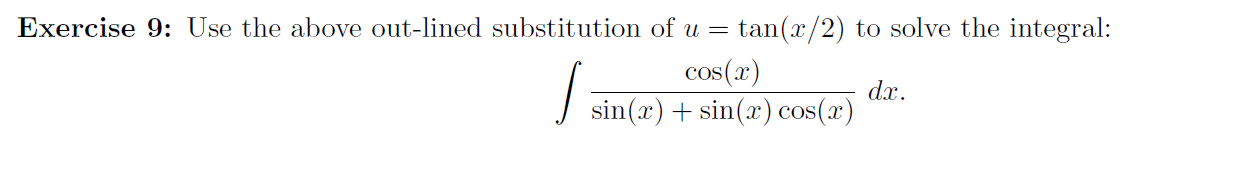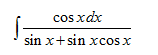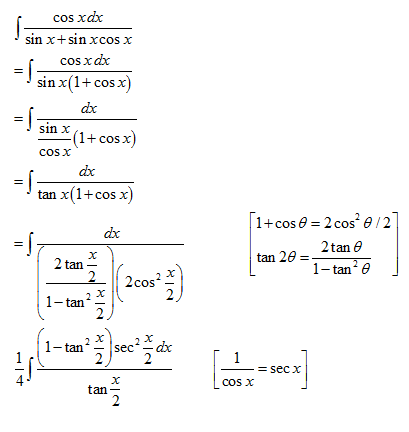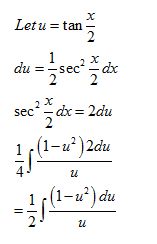# Exercise 9: Use the above out-lined substitution of u = tan(x/2) to solve the integral:cos(x)J sin(x) + sin(x) cos(x)dx.

Question
1 viewshelp_outlineImage TranscriptioncloseExercise 9: Use the above out-lined substitution of u = tan(x/2) to solve the integral: cos(x) J sin(x) + sin(x) cos(x) dx. fullscreen
check_circle

Step 1Step 2Step 3...

### Want to see the full answer?

See Solution

#### Want to see this answer and more?

Solutions are written by subject experts who are available 24/7. Questions are typically answered within 1 hour.*

See Solution
*Response times may vary by subject and question.
Tagged in